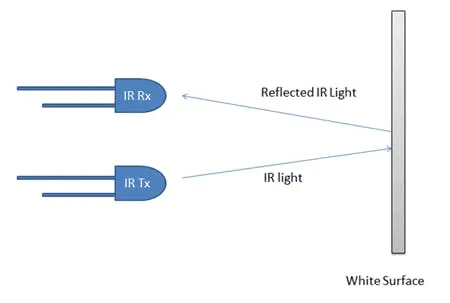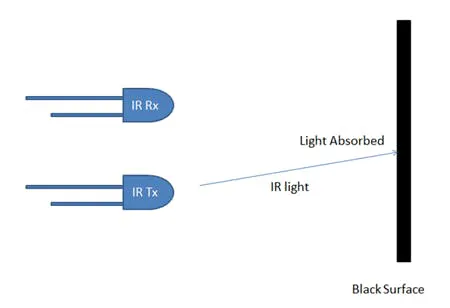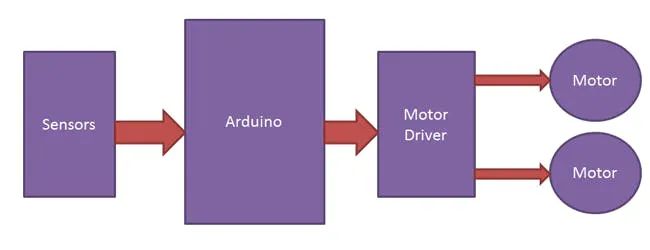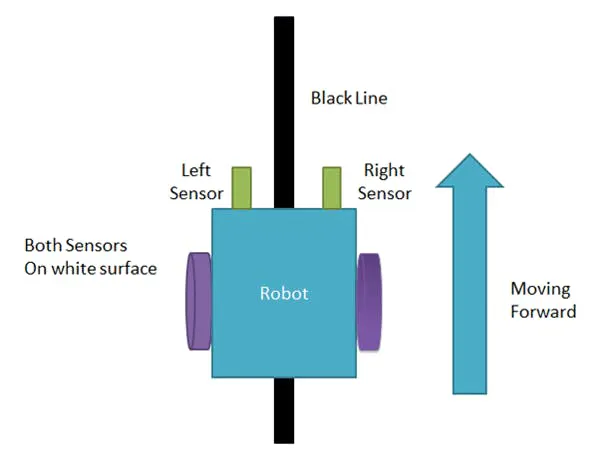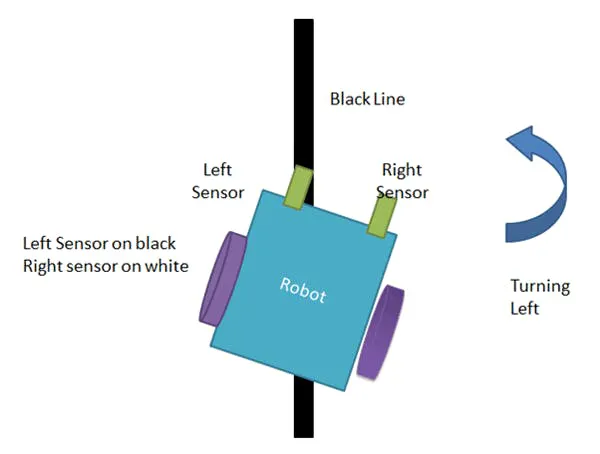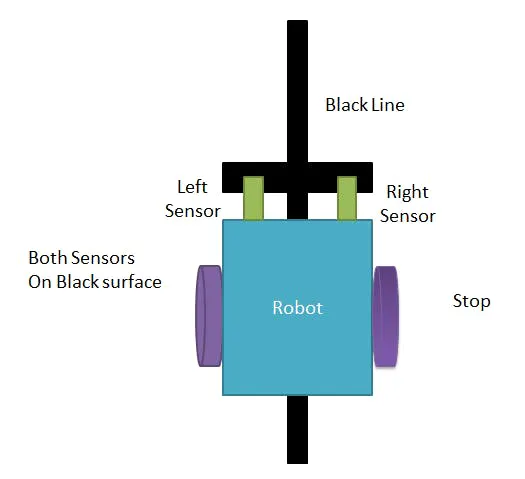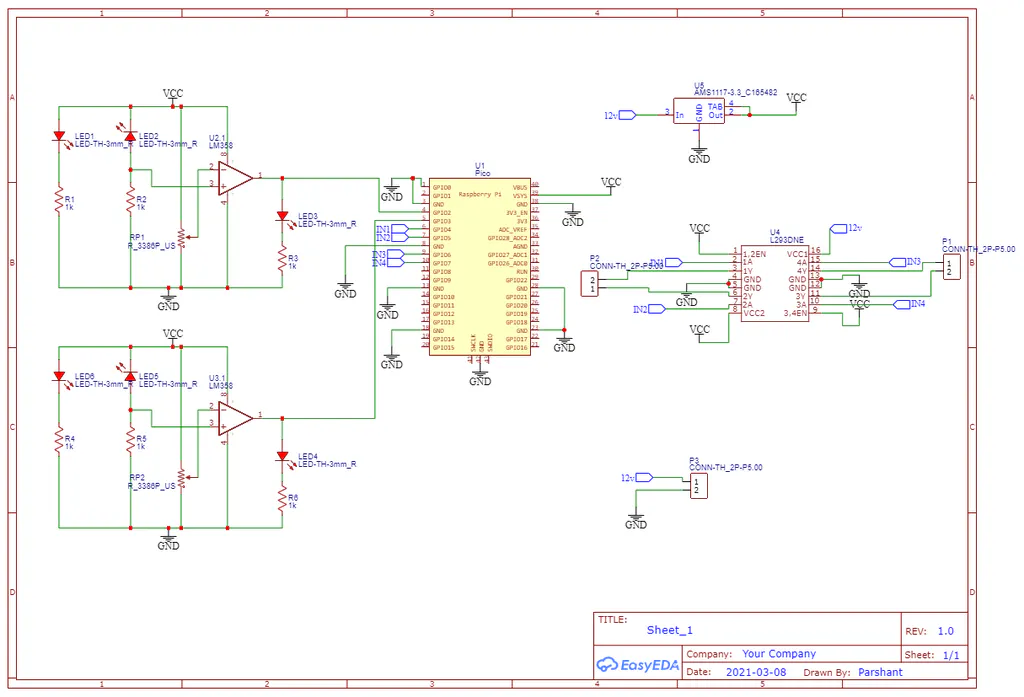from machine import Pin
import time

in1 = Pin(2, Pin.IN)
in2 = Pin(3, Pin.IN)

m11 = Pin(4, Pin.OUT)
m12 = Pin(5, Pin.OUT)
m21 = Pin(6, Pin.OUT)
m22 = Pin(7, Pin.OUT)

while True:
sensor1 = in1.value()
sensor2 = in2.value()
if sensor1 == 1:
if sensor2 == 1:
m11.value(0)
m12.value(1)
m21.value(0)
m22.value(1)
elif sensor2 == 0:
m11.value(0)
m12.value(0)
m21.value(0)
m22.value(1)
elif sensor1 == 0:
if sensor2 == 1:
m11.value(0)
m12.value(1)
m21.value(0)
m22.value(0)
else:
m11.value(0)
m12.value(0)
m12.value(0)
m22.value(0)

LFR沿黑线，将其编程为当两个传感器都变为黑色时停止工作。因此，在纸张的这一侧，它在每个交叉点处停止。加入微信技术交流群关注与非网服务号加入电路城 QQ 交流群